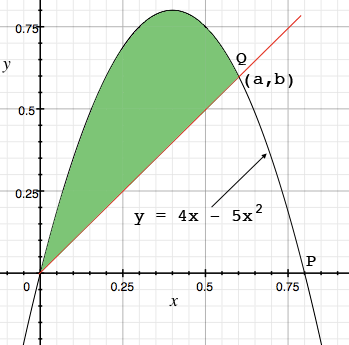SEARCH HOMEMath Central Quandaries & QueriesQuestion from Mandy: There is a line through the origin that divides the region bounded by the parabola y=4x−5x^2 and the x-axis into two regions with equal area. What is the slope of that line?Hi Mandy,

I drew a graph of $y = 4x - 5x^2 = x(4 - 5x)$ and a line through the origin that looks like it divides the area between the graph and the X-axis in half. This line intersects the graph at the origin and Q which has coordinates $(a,b).$ This line then has slope $\large \frac{b}{a}.$I would first integrate $y = 4x - 5x^2$ from $0$ to $P$ to find the area between the graph and the X-axis. Call this area $B.$

Next find the area shaded green in the diagram, again by integration. Set this area equal to $\large \frac{B}{2}$ and solve for $a.$

Write back if you need more assistance,
Penny* Registered trade mark of Imperial Oil Limited. Used under license.Math Central is supported by the University of Regina and the Imperial Oil Foundation.